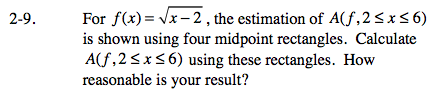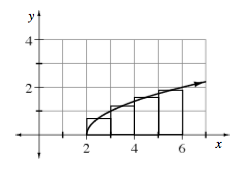### Home > CALC > Chapter Ch2 > Lesson 2.1.1 > Problem2-9

2-9.A = (base)(height)
Base = 1 for all rectangles. Height is determined by the function, evaluated at midpoints.

$\text{The height of the smallest rectangle is }\sqrt{0.5}.$

$\text{The height of the largest rectangle is }\sqrt{3.5}.$